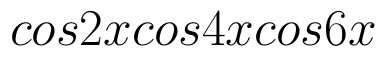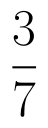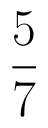Select Page

# Solutions for MCQ CBSE 12 Science Maths Application of Integrals in English

Solutions for MCQ CBSE 12 Science Maths Application of Integrals in English to enable students to get Solutions in a narrative video format for the specific question.

Expert Teacher provides Solutions for MCQ CBSE 12 Science Maths Application of Integrals through Video Solutions in English language. This video solution will be useful for students to understand how to write an answer in exam in order to score more marks. This teacher uses a narrative style for a question from Application of Integrals not only to explain the proper method of answering question, but deriving right answer too.

Please find the question below and view the Solution in a narrative video format.

Question:

Solution Video in English:

## Similar Questions from CBSE, 12th Science, Maths, Application of Integrals

Question 1 :

Find the area of the given curves and given lines:and x-axis

Question 2 : Find the area of the region. (View Answer Video)

Question 3 : Using integration, find the area of theco-ordinates whose vertices are P(2,0), Q(4, 5) and R(6,3). (View Answer Video)

Question 4 : Find the area bounded by curvesand(View Answer Video)

Question 5 : Find the area enclosed by the parabolaand the line x - y = 4. (View Answer Video)

### Determinant

Question 1 : Evaluate. (View Answer Video)

Question 2 : The solution of the following system of equation is 2x + 3y = 5, 5x – 2y = 3. (View Answer Video)

Question 4 :  Evaluate the determinants:. (View Answer Video)

Question 5 : Evaluate the determinant:. (View Answer Video)

### Integrals

Question 1 :  Find the integral of the function. (View Answer Video)

Question 2 : Find :(View Answer Video)

Question 3 : Evaluate :(View Answer Video)

Question 4 : Prove that :(View Answer Video)

Question 5 : Given. Write f(x) satisfying the above.  (View Answer Video)

### Probability

Question 1 : In a game, a man wins rupee five for number six and losses rupee one for any other number, when a fair die is thrown. The man decided to throw a die thrice but to quit as and when he gets a six. Find the expected value of the amount he wins/losses. (View Answer Video)

Question 2 : There are three coins. First is a biased that comes up tails 60% of the times, second is also a biased coin that comes up heads 75% of the times and third is an unbiased coin. One of the three coins is chosen at random and tossed, it shows heads, what is the probability that it was the first coin?      (View Answer Video)

Question 3 : The probabilities of two students A and B coming to the school in time areandrespectively. Assuming that the events ' A coming in time ' and
' B coming in time ' are independent. Find the probability of only one. Write of them come in time atleast one advantage of coming to school in time.   (View Answer Video)

Question 4 : A and B throw a pair of dice alternately. A wins the game if he gets a total of 7 and B wins the game if he gets a total of 10. If A starts the game, the find the probability that B wins.   (View Answer Video)

Question 5 : A pair of dice is thrown 4 times. If getting a doublet is considered a success, find the probability distribution of the number of successes and hence find its mean.  (View Answer Video)